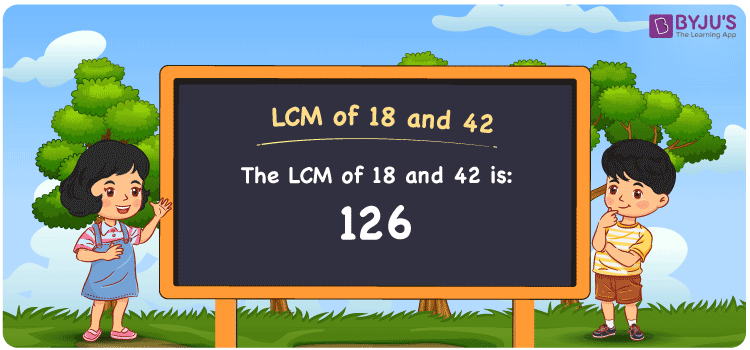# LCM of 18 and 42

LCM of 18 and 42 is 126. LCM, stands for Least Common Multiple or Lowest common multiple. It is the smallest or the first positive number that can be divided by the given set of numbers. Consider the example for finding the LCM of 18 and 42. The answer is 126. 126 is divisible by both 18 and 42. Even 252 is divisible by 18 and 42, however it is not the LCM for 18 and 42. The smaller number than 252 is 126 which is divisible by both 18 and 42. Hence 126 is the Least Common Multiple for 18 and 42. You can use the Prime Factorization and Division method to find the LCM of any 2 numbers.

## What is LCM of 18 and 42

The Least Common Multiple or Lowest Common Multiple of 18 and 42 is 126.## How to Find LCM of 18 and 42?

LCM of 18 and 42 can be determined using three methods:

• Prime Factorisation
• Division method
• Listing the multiples

### LCM of 18 and 42 Using Prime Factorisation Method

In the Prime Factorisation method for finding the LCM of 18 and 42, the numbers 18 and 42 are expressed as the multiples of prime numbers.

18 = 2 × 3 × 3

42 = 2 × 3 × 7

LCM (18, 42) = 2 × 3 × 3 × 7 = 126

### LCM of 18 and 42 Using Division Method

In the Division Method for finding the LCM of 18 and 42, the numbers are divided with the smallest number and continued till 1 remains as the remainder.

 2 18 42 3 9 21 3 3 7 7 1 7 × 1 1

LCM (18, 42) = 2 × 3 × 3 × 7 = 126

### LCM of 18 and 42 Using Listing the Multiples

By listing all the multiples of given numbers, we can identify the first number that is common multiples in both the tables. Below is the list of multiples for 18 and 42

 Multiples of 18 Multiples of 42 18 42 36 84 54 126 72 168 90 210 108 252 126 294 144 336

LCM (18, 42) = 126

## Video Lesson on Applications of LCM## Solved Examples

What is the smallest number that is divisible by both 18 and 42?

Answer: 126 is the smallest number that is divisible by both 18 and 42.

What is the LCM for 2, 6, 18 and 42?

Answer: LCM for 2, 6, 18 and 42 is 126 as 2 and 6 are also the factors of 18 and 42.

## Frequently Asked Questions on LCM of 18 and 42

### What is the LCM of 18 and 42?

The LCM of 18 and 42 is 126.

### Is the LCM of 18 and 42 same as the HCF of 18 and 42?

No. Least Common Multiple of 18 and 42 is 126 and the HIghest Common Factor of 18 and 42 is 6.

### Is the LCM and LCD both the same for 18 and 42?

Yes. The Least Common Multiple and Lowest Common Divisor are both the same for 18 and 42, which is 126.

### What are the first 3 common multiples of 18 and 42?

The first three common multiples of 18 and 42 are 126, 252  and 378.

### Is the LCM of 9 and 18 the same as the LCM of 9 and 42?

No. LCM of 9 and 18 is 18, whereas the LCM of 9 and 42 is 126. They are not the same.